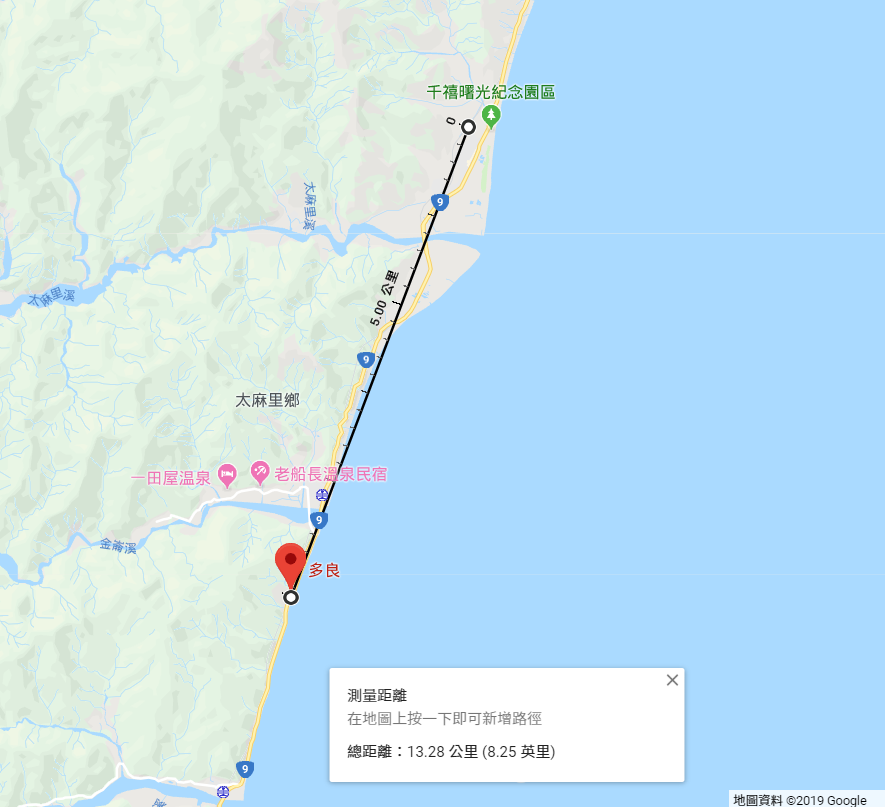#DAY 23
0
AI & Data

## ST_DWithin()

``````SELECT subid, kind, bodytype, colour, age,
WHERE ST_DWithin(geog_point,
ST_GeogFromText('POINT(120.9 22.5)')),
1000)
ORDER BY subid;
``````

## ST_Distance() 算出距離

`ST_Distance()`方法會算出兩點之間的距離，我們先來隨意計算兩個點。

``````SELECT ST_Distance(
ST_GeogFromText('POINT(120.959186 22.507383)'),
ST_GeogFromText('POINT(121.004909 22.619066)')
)/1000;

-- "13.23142074176"
``````## 最後

``````SELECT  subid,
kind,
bodytype,
colour,
age,
round(
(ST_Distance(geog_point,
ST_GeogFromText('POINT(120.959186 22.507383)')
) / 15000)::numeric(8,5), 2
) AS km_from_station
WHERE ST_DWithin(geog_point,
ST_GeogFromText('POINT(120.959186 22.507383)'),
15000)
ORDER BY km_from_station ASC;
``````